Figure 18.1 Two images of the Moon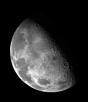R code
 Figure 18.2 Adding an imported image to a plot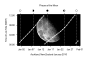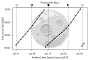R code
 Figure 18.3 Two raster images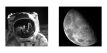R code
 Figure 18.4 Manipulating raster imagesR code
 Figure 18.5 Breaking a vector image into paths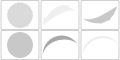R code
 Figure 18.6 Variations on the Moon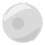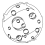R code